• 利用mathematica计算复数的对数难以找到合适的帮助文档，有意思的是找了基本数学手册居然没有复数对数的普遍运算公式，真是奇怪。不得不自己动手弄出来，本人将复数的各种运算包括指数特别是对数的运算方法和技巧写...
• MATLAB笔记之复数基本公式 QQ:3020889729 小蔡复数的创建复数的实部与虚部的获取复数的辐角与模的获取复数的共轭运算 QQ:30...


MATLAB笔记之复数基本公式
QQ:3020889729                                                                                 小蔡复数的创建复数的实部与虚部的获取复数的辐角与模的获取复数的共轭运算

QQ:3020889729                                                                                 小蔡
复数的创建
% 创建复数
z1 = 2 + 7*1i;% 表达式直接创建
z2 = 2 + 7*i;% 注意了，有时候版本要求，提示需要将*1i代替i来避免错误
z3 = complex(2.7);% 创建实部为2，虚部为7的的复数

效果：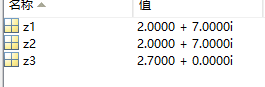复数的实部与虚部的获取
% 复数的实部与虚部的获取
z0 = complex(2,7);% 创建实部为2，虚部为7的的复数
z0_Im = imag(z0);% 用imag获取复数的虚部
z0_Re = real(z0);% 用real获取复数的实部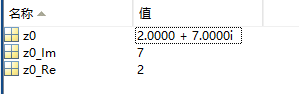复数的辐角与模的获取
% 复数的辐角与模的获取
z0 = complex(2,7);% 创建实部为2，虚部为7的的复数
z0_Mod = abs(z0);% 用abs获取复数的模——也可以用来求实数的绝对值
z0_Arg = angle(z0);% 用angle获取复数的辐角（主）值——也可以求其它实数的相位角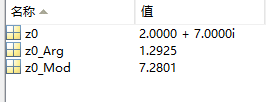复数的共轭运算
% 复数的共轭运算
clear,clc;
z0 = complex(2,7);% 创建实部为2，虚部为7的的复数
z1 = conj(z0);% 取得指定复数的(复)共轭复数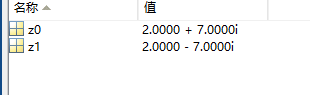展开全文matlab
• ## 复数的运算法则

千次阅读 2021-01-21 14:52:27
负数的运算包括加法法则，乘法法则，除法法则，开方法bai则，运算律，i的乘方法则等。具体运算方法如下： 1.加法法则 复数的加法法则：设z1=a+bi，z2=c+di是任意两个复数。两者和的实部是原来两个复数实部的和，它的...
负数的运算包括加法法则，乘法法则，除法法则，开方法bai则，运算律，i的乘方法则等。具体运算方法如下：
1.加法法则
复数的加法法则：设z1=a+bi，z2=c+di是任意两个复数。两者和的实部是原来两个复数实部的和，它的虚部是原来两个虚部的和。两个复数的和依然是复数。即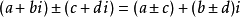2.乘法法则
复数的乘法法则：把两个复数相乘，类似两个多项式相乘，结果中i2= -1，把实部与虚部分别合并。两个复数的积仍然是一个复数。即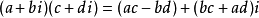3.除法法则
复数除法定义：满足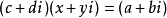的复数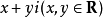叫复数a+bi除以复数c+di的商。
运算方法：将分子和分母同时乘以分母的共轭复数，再用乘法法则运算，
即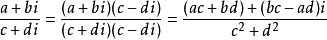4.开方法则
若zn=r(cosθ+isinθ），则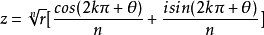（k=0，1，2，3…n-1）
5.运算律
加法交换律：z1+z2=z2+z1
乘法交换律：z1×z2=z2×z1
加法结合律：(z1+z2)+z3=z1+(z2+z3)
乘法结合律：(z1×z2)×z3=z1×(z2×z3)
分配律：z1×(z2+z3)=z1×z2+z1×z3
6.i的乘方法则
i4n+1=i, i4n+2=-1, i4n+3=-i, i4n=1（其中n∈Z）
7.棣莫佛定理
对于复数z=r(cosθ+isinθ），有z的n次幂
zn=rn[cos(nθ)+isin(nθ)]　（其中n是正整数）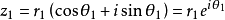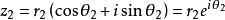则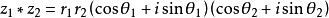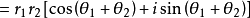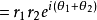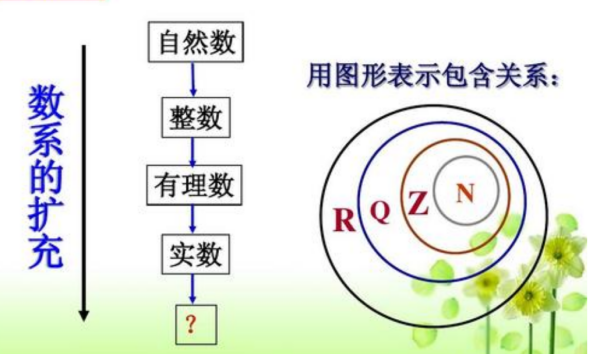参考资料: 复数如何运算. 百度百科–复数
展开全文抽象代数
• 笔记


z

=

x

+

i

y

z=x+iy

arg

⁡

z

\arg z

x

<

0

x<0

x

=

0

x=0

x

>

0

x>0

y

<

0

y<0

arctan

⁡

y

x

−

π

\arctan\frac{y}{x}-\pi

−

1

2

π

-\frac{1}{2}\pi

arctan

⁡

y

x

\arctan\frac{y}{x}

y

=

0

y=0

π

\pi

0

0

y

>

0

y>0

arctan

⁡

y

x

+

π

\arctan\frac{y}{x}+\pi

1

2

π

\frac{1}{2}\pi

arctan

⁡

y

x

\arctan\frac{y}{x}

2021年3月21日19:32:16
展开全文算法 线性代数
• 这个展开式式需要了解相关知识，这里给出欧拉公式，主要是为电路中的相量法而服务的！
这个展开式式需要了解相关知识，这里给出欧拉公式，主要是为电路中的相量法而服务的！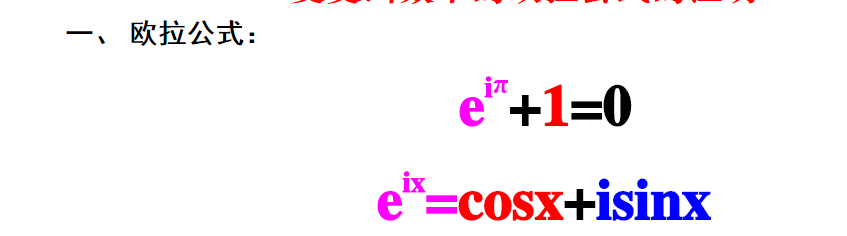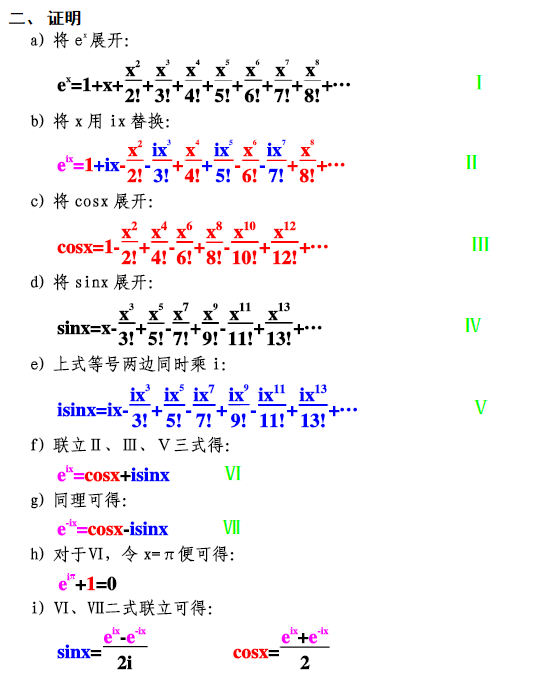展开全文• 实变函数（高等数学）主要内容： ...一、复数基本知识 1.1 复数基本概念 对任意两实数x, y，称z=x+iyz=x+iyz=x+iy或z=x+yiz=x+yiz=x+yi为复数，其中i2=−1i^2=-1i2=−1，i称为虚部 复数z的实部Re(z)=x，复变函数
• 欧拉复数公式欧拉复数公式欧拉公式泰勒级数展开近似值 欧拉复数公式 公式： eiπ+1=0e^{i\pi}+1 = 0eiπ+1=0 这个方程真的很奇妙，因为它集合了： eee （欧拉数） iii （单位 虚数) π\piπ （大名鼎鼎的 pi，一个...欧拉回路 latex 代码规范
• 选择实数或者复数进行运算，手动输入数据，然后进行加减乘除操作运算，输出结果
• 数学物理方法·基础③复数基本运算法则 QQ:3020889729 小蔡复数运算规律复数加法运算复数乘法运算复数除法运算复数乘方运算复数开方运算(使用欧拉方程更方便) ...
• 数学 库，带有使用Python处理数学函数和公式的工具。Python
• ## Java实现复数运算

万次阅读 多人点赞 2019-07-21 21:44:59
设有两个复数 和 ，则他们的运算公式为： 要求：（1）定义一个结构体类型来描述复数。 　（2）复数之间的加法、减法、乘法和除法分别用不用的函数来实现。 　（3）必须使用结构体指针的方法把函数的计算结果返回。 ...Java 复数
• c语言进行虚数的四则运算 额。。好像没什么好说的直接上代码吧，注意下结构体的用法 #include<stdio.h> struct complex { double re; double im; }; complex add(complex x,complex y); complex ...
•线性代数
• 复数运算法则： 加法运算复数的加法按照以下规定的法则进行：设z1=a+bi,z2=c+di是任意两个复数，则它们的和是(a+bi)+(c+di)=(a+c)+(b+d)i; 例如：a = 1+2i,b = 3+4i 即可得 a+b = 4+6i 减法法则： 复数的减法...
• 转自：https://blog.csdn.net/qq_40724865/article/details/78556918 转载于:https://www.cnblogs.com/yuankai-ren/p/11606390.html
• ## 欧拉公式，复数域的成人礼

千次阅读 多人点赞 2018-12-19 14:17:30
之前在“复数，通往真理的最短路径”中说过，复数域其实就是二维的数域，提供了更高维度的、更抽象的视角。本文来看看，我们是怎么从实数域扩展到复数域的。 大家可能觉得这个扩展并不复杂，也就是 、 两个任意...
• （这将是百度上关于复数运算较为全面的一篇） 加法 (a+bi)+(c+di)=(a+c)+(b+d)i 减法 (a+bi)+(c+di)=(a-c)+(b-d)i 乘法 (a+bi)(c+di)=(ac-bd)+(bc+ad)i 除法 (a+bi)/(c+di)=(ac+bd)/(c ^ 2 + d...复变函数 复数对数
• //复对数运算 public class fushu2 { static void cLog(double a,double b,double[] e,double[] f){ double temp; temp=Math.log(Math.sqrt(a*a+b*b)); e=temp; f=Math.atan2(b,a); } //复指数运算e的(a+bi)次...java
• ## c++之复数类运算

万次阅读 多人点赞 2016-07-06 14:53:18
那么我们大体回顾一下复数，即a+bi,那么关于它的运算法则大体有以下几种。 1.加法法则  复数的加法按照以下规定的法则进行：设z1=a+bi,z2=c+di是任意两个复数，则它们的和是  (a+bi)+(c+di)=(a+c)+(b+d)i;...c++ 函数重载
• 复数： z = x + jy. 通常在平面坐标中表示， x轴为实轴， y轴为虚轴。 复数加法和减法是实轴和实轴相加，虚轴和虚轴相加。 乘法： 欧拉公式： e^iθ = cos(θ) + j sin(θ) 当 θ = π ， e^iπ = -1 ...
• 式中sgn表示符号函数，即sng(x)={1 if x>00 if x=0−1 if x<0sng(x)= \begin{cases} 1 & \text{ if } x>0 \\ 0 & \text{ if } x=0 \\ -1 &...x.
• 设有两个复数 和 ，则他们的运算公式为： 　要求：（1）定义一个结构体类型来描述复数。 　（2）复数之间的加法、减法、乘法和除法分别用不用的函数来实现。 　（3）必须使用结构体指针的方法把函数的计算结果返回。...
• 复数的幂运算 根据欧拉公式有： 根据De Moivre' law有： 示例： 转载于:https://www.cnblogs.com/liuyongdun/p/6955160.html
• 设有两个复数 和 ，则他们的运算公式为： 要求：（1）定义一个结构体类型来描述复数。 　（2）复数之间的加法、减法、乘法和除法分别用不用的函数来实现。 　（3）必须使用结构体指针的方法把函数的计算结果返回。 ...java
• 韶 关 学 院 学 生 实 验 报 告 册 实验课程名称数据结构与算法 实验项目名称实验一 抽象数据类型 复数四则运算 实验类型 打 基础 综合 设计 院 系信息工程学院计算机系 专 业* 姓 名* 学 号* 指导老师陈正铭 韶关...
• 设有两个复数 和 ，则他们的运算公式为： 要求：（1）定义一个结构体类型来描述复数。 　（2）复数之间的加法、减法、乘法和除法分别用不用的函数来实现。 　（3）必须使用结构体指针的方法把函数的计算结果返回。 ...
• 在之后的项目中有编写复数函数的要求，所以先总结资料以备用。同时也发布在这里以供大家参考。
• 向量、矩阵、实数、复数之间的各种运算方法 1.复数的乘法 复数 z1 = a + bi。 复数 z2 = c + di。 z1和z2的乘积：(ac-bd)+(bc+ad)i。 以下为代码实现： //复数结构体定义 typedef struct { float ......